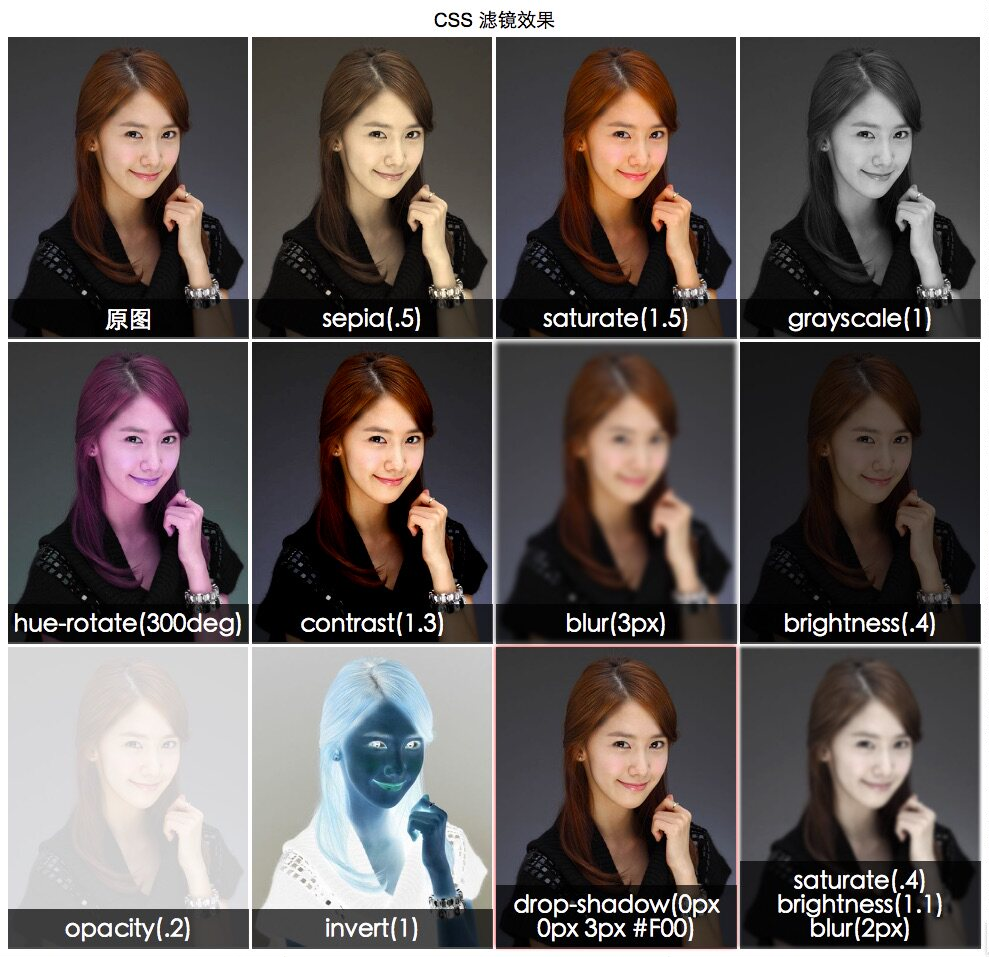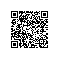# canvas位图处理

HTML5 canvas为我们提供了一块画布，让前端也有了操作位图的功能：图片旋转、缩放、滤镜、压缩等都可以通过JS来实现。

### 图像滤镜处理

// 复古滤镜处理算法：获取每个像素的RGB信息，并按特定权重返回其加权平均值
let sepiaFilter = function(imgData) {
let d = imgData.data
for (let i = 0; i < d.length; i += 4) {
let r = d[i]
let g = d[i + 1]
let b = d[i + 2]
d[i] = (r * 0.393) + (g * 0.769) + (b * 0.189) // red
d[i + 1] = (r * 0.349) + (g * 0.686) + (b * 0.168) // green
d[i + 2] = (r * 0.272) + (g * 0.534) + (b * 0.131) // blue
}
return imgData
}
//图像地址必须和当前页面同域，否则会报cross-origin错误
img.src = '/img/logo@2x.png'
ctx.drawImage(img, 0, 0) // 绘制原图
let imgData = ctx.getImageData(0, 0, img.width, img.height) // 获取图片信息
ctx.putImageData(sepiaFilter(imgData), 100, 0) // 绘制处理后图片
}

### 图像base64存储

// 支持三种文件类型：image/png(默认) | image/jpeg | image/webp(仅Chrome)
canvas.toDataURL() // 默认存储为png
// 仅jpeg/webp支持质量参数(0~1,默认0.92)
canvas.toDataURL("image/jpeg", 0.1) // 存储为质量为0.1的jpeg

### 程序员的备胎

注意：本人很专一。

#### AlloyImage# SVG矢量图处理

SVG遵循XML规范，可以很好的集成在HTML里面，同时支持JS脚本控制，还有基于SMIL标准的动态内容支持，做起动画来也是非常方便。目前基于SVG的JS图形库轮子也是非常多，如svg.js, Snap.svg, Velocity.js, D3.js等等，目前暂无SVG应用需求，等用的时候再翻牌子吧。

# CSS图像处理# 总结使用钉钉扫一扫加入圈子
+ 订阅